# Introduction

pavo is an R package developed with the goal of establishing a flexible and integrated workflow for working with spectral and spatial color data. It includes functions that take advantage of new data classes to work seamlessly from importing raw spectra and images, to visualization and analysis. It provides flexible ways to input spectral data from a variety of equipment manufacturers, process these data, extract variables, and produce publication-quality figures.

pavo was written with the following workflow in mind:

1. Organize data by importing and processing spectral and image data (e.g., to remove noise, negative values, smooth curves, etc.).
2. Analyze the resulting files, using spectral analyses of shape (hue, saturation, brightness), visual models based on perceptual data, and/or spatial adjacency and boundary strength analyses.
3. Visualize the output, with multiple options provided for exploration and analysis.

Below we will show the main functions in the package in example workflows. The development version of pavo can be found on github.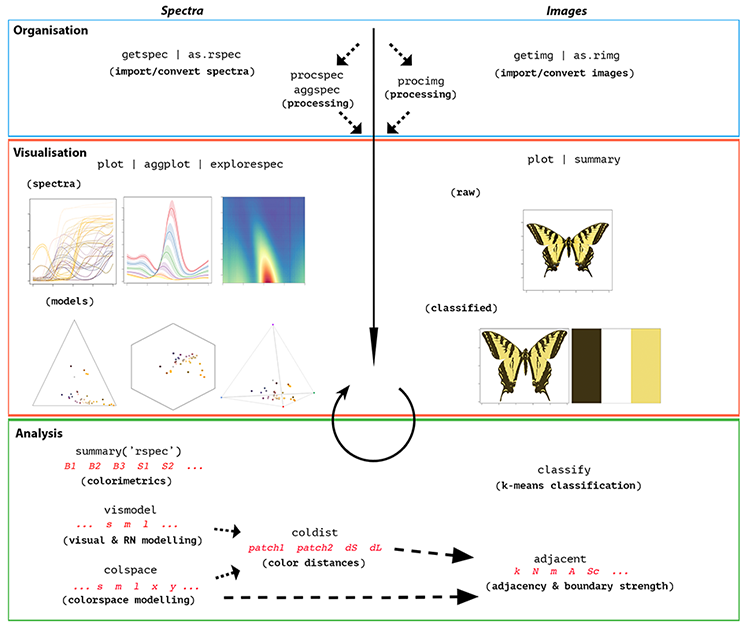# Load the package, and set a global random-number seed for the reproducible generation of fake data later on.
library(pavo)
set.seed(1612217)


# Organizing Spectral Data

## Spectral Dataset Description

The raw spectral data used in this example are available from the package reporitory on github, located here here. You can download and extract it to follow the vignette exactly. Alternately, the data are included as an RData file as part of the package installation, and so can be loaded directly (see below).

The data consist of reflectance spectra, obtained using Avantes equipment and software, from seven bird species: Northern Cardinal Cardinalis cardinalis, Wattled Jacana Jacana jacana, Baltimore Oriole Icterus galbula, Peach-fronted Parakeet Aratinga aurea, American Robin Turdus migratorius, and Sayaca Tanager Thraupis sayaca. Several individuals were measured (sample size varies by species), and 3 spectra were collected from each individual. However, the number of individuals measured per species is uneven and the data have additional peculiarities that should emphasize the flexibility pavo offers, as we'll see below.

In addition, pavo includes three datasets that can be called with the data function. data(teal), data(sicalis), and data(flowers) will all be used in this vignette. See help for more information help(package = "pavo").

## Importing

The first thing we need to do is import spectral data into R using the function getspec(). Since the example spectra were obtained using Avantes software, we will need to specify that the files have the .ttt extension. Further, the data is organized in subdirectories for each species. getspec does recursive sampling, and may include the names of the subdirectories in the spectra name if desired. getspec also uses parallel processing when the argument cores is set to a number greater than 1 (currently available only on Linux and Mac). A final issue with the data is that it was collected using a computer with international numbering input, which means it uses commas instead of periods as a decimal separator. We can specify that in the function call.

If, for example, the raw spectral files were downloaded and placed in a directory called /pavo/vignette_data, you might execute the following command and see this output:

specs <- getspec("~/pavo/vignette_data/", ext = "ttt", decimal = ",", subdir = TRUE, subdir.names = FALSE)
# 213  files found; importing spectra
# |================================================================================| 100%, ETA 00:00


For convenience, however, we've included the spectra as an RData file in the package installation, and so will simply load it directly.

specs <- readRDS(system.file("extdata/specsdata.rda", package = "pavo"))


And we can inspect the resulting object:

specs[1:10, 1:4]

##     wl cardinal.0001 cardinal.0002 cardinal.0003
## 1  300        5.7453        8.0612        8.0723
## 2  301        6.0181        8.3926        8.8669
## 3  302        5.9820        8.8280        9.0680
## 4  303        6.2916        8.7621        8.7877
## 5  304        6.6277        8.6819        9.3450
## 6  305        6.3347        9.6016        9.4834
## 7  306        6.3189        9.5712        9.3533
## 8  307        6.7951        9.4650        9.9492
## 9  308        7.0758        9.4677        9.8587
## 10 309        7.2126       10.6172       10.5396

dim(specs) # the data set has 213 spectra, from 300 to 700 nm, plus a 'wl' column

##  401 214


When pavo imports spectra, it creates an object of class rspec, which inherits attributes from the data.frame class:

is.rspec(specs)

##  TRUE


If you already have multiple spectra in a single data frame that you'd like to use with pavo functions, you can use the command as.rspec to convert it to an rspec object. The function will attempt to identify the wavelength variable or you can specify the column containing wavelengths with the whichwl argument. The default way that as.rspec handles reflectance data is to interpolate the data in 1-nm bins, as is commonly done for spectral analyses. However, this can be turned off by using: interp = FALSE. As an example, we will create some fake reflectance data, name the column containing wavelengths (in 0.5-nm bins) wavelength rather than wl (required for pavo functions to work) and also put the column containing wavelengths third rather than first.

# Create some fake reflectance data with wavelength column arbitrarily titled
# and not first in the data frame:
fakedat <- data.frame(
refl1 = rnorm(n = 801),
refl2 = rnorm(n = 801),
wavelength = seq(300, 700, by = .5)
)

##          refl1      refl2 wavelength
## 1 -0.032893386  0.5059612      300.0
## 2 -0.478552738 -1.1526035      300.5
## 3 -0.190687886 -1.0708952      301.0
## 4 -0.008977959 -1.9871907      301.5
## 5 -0.443133039 -0.3910143      302.0
## 6  0.032110206 -0.5403221      302.5

is.rspec(fakedat)

##  FALSE

fakedat.new <- as.rspec(fakedat)

## wavelengths found in column 3

is.rspec(fakedat.new)

##  TRUE

head(fakedat.new)

##    wl       refl1       refl2
## 1 300 -0.03289339  0.50596119
## 2 301 -0.19068789 -1.07089518
## 3 302 -0.44313304 -0.39101435
## 4 303 -0.52064707  0.84403232
## 5 304  1.42813472  0.07150436
## 6 305 -0.19044804 -0.84504226


As can be seen, as.rspec renames the column containing wavelengths, sets it as the first column, interpolates the data in 1-nm bins and converts the data to an rspec object. Note that the same output is returned with specifying whichwl = 3:

head(as.rspec(fakedat, whichwl = 3))

##    wl       refl1       refl2
## 1 300 -0.03289339  0.50596119
## 2 301 -0.19068789 -1.07089518
## 3 302 -0.44313304 -0.39101435
## 4 303 -0.52064707  0.84403232
## 5 304  1.42813472  0.07150436
## 6 305 -0.19044804 -0.84504226


Finally, the lim argument allows you to specify the range of wavelengths contained in the input dataset. This is useful either in the case that the dataset doesn't contain this information (and hence you cannot specify the column with whichwl or automatically find the column with as.rspec). Additionally, it may be useful to focus on a subset of wavelength. In our example, the wavelengths ranged from 300 to 700 nm, however you could also specify a restricted range of wavelengths with lim:

fakedat.new2 <- as.rspec(fakedat, lim = c(300, 500))

## wavelengths found in column 3

plot(fakedat.new2[, 2] ~ fakedat.new2[, 1], type = "l", xlab = "wl")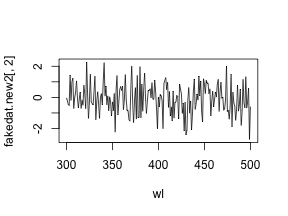We want to stress that it is important to check the actual wavelengths contained in the data before setting this argument (as.rspec will warn you when wavelengths in the data are not present in the range specified with lim), otherwise as.rspec will assume that wavelengths exist when in fact they may not. For example, if we set lim = c(300, 1000) and plot the results, the reflectance values between 700 and 1000 nm are set to be equal since there is no information at these wavelengths in the original dataset:

fakedat.new2 <- as.rspec(fakedat, lim = c(300, 1000))

## wavelengths found in column 3

## Warning in as.rspec(fakedat, lim = c(300, 1000)): Specified wavelength
## limits outside of actual data. Check 'lim' argument.

plot(fakedat.new2[, 2] ~ fakedat.new2[, 1], type = "l")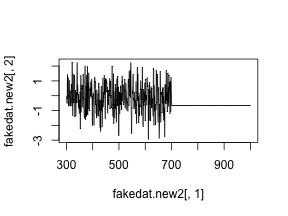## Subsetting and Merging Spectral Data

Once an rspec object has been created, either by importing raw spectral data or converting a dataset with the as.rspec function, you can subset the spectra based on their names using a modified version of R's built-in subset function. For example, the following code illustrates how to create an rspec object containing only tanagers:

specs.tanager1 <- subset(specs, "tanager")


##    wl tanager.0001 tanager.0002 tanager.0003 tanager.0004
## 1 300      10.0618      10.6744      10.1499      13.7473
## 2 301      11.1472      10.8054       9.8003      14.3102
## 3 302      10.7819      10.6134       9.5607      14.4463
## 4 303      11.0210      11.2037      10.4107      15.5533
## 5 304      10.2177      11.2120       9.9452      14.3841
## 6 305      11.5664      11.6135      10.8659      15.6445


The subset function here is using partial matching to find all spectra with the string “tanager” in their name. To fully benefit from this flexible subsetting functionality, it is important that you follow a consistent file naming scheme. For example, tanager.14423.belly.001.ttt would indicate the species (tanager), individual ID (14423), body patch (belly) and measurement number (001). Additionally, we suggest that labels used should have the same number of characters, which simplifies character string manipulation and subsetting based on partial matching.

If you prefer not to use partial matching, subset will also work if you provide a logical condition, similar to the default subset behavior in R. For example:

# extract first component of filenames containing species names
spp <- do.call(rbind, strsplit(names(specs), "\\."))[, 1]

# subset
specs.tanager2 <- subset(specs, subset = spp == "tanager")

# compare subsetting methods
all.equal(specs.tanager1, specs.tanager2)

##  TRUE


Note that subset will also work with visual model (class vismodel) and colspace (class colspace) objects, as described below.

Another useful function is merge. Let's say that you have subsetted spectra for tanagers and parakeets, and you would like to re-combine them for an analysis. The following lines of code show how to do this:

specs.tanager <- subset(specs, "tanager")
specs.parakeet <- subset(specs, "parakeet")
specs.new <- merge(specs.tanager, specs.parakeet)


Note that this re-combined file (specs.new) has only a single wl column with the merged spectra as columns. Keep in mind that the order of objects in merge will determine the order of columns in the final merged object (i.e. tanagers will be before parakeets).

# Processing Spectral Data

## Averaging Spectra

As previously described, our data (contained in the specs object) constitutes of multiple individuals, and each was measured three times, as is common to avoid measurement bias. A good way to visualize the repeatability of our measurements is to plot the spectra of each individual separately. The function explorespec provides an easy way of doing so. You may specify the number of spectra to be plotted in the same panel using the argument specreps, and the function will adjust the number of panels per page accordingly. We will exemplify this function using only the 12 cardinal individuals measured:

# 36 spectra plus the first (wl) column
explorespec(specs[, 1:37], by = 3, lwd = 2)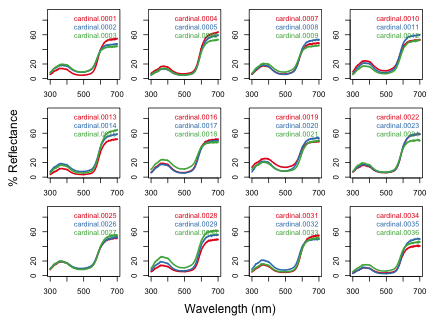So our first step would be to take the average of each of these three measurements to obtain average individual spectra to be used in further analyses. This is easily accomplished using the aggspec function. The by argument can be either a number (specifying how many specs should be averaged for each new sample) or a vector specifying the identities of the spectra to be combined (see below):

mspecs <- aggspec(specs, by = 3, FUN = mean)
mspecs[1:5, 1:4]

##    wl cardinal cardinal.1 cardinal.2
## 1 300 7.292933   5.676700   6.387233
## 2 301 7.759200   5.806700   6.698200
## 3 302 7.959333   5.858467   6.910500
## 4 303 7.947133   6.130267   7.357567
## 5 304 8.218200   6.127933   7.195267

dim(mspecs) # data now has 71 spectra, one for each individual, and the 'wl' column

##  401  72


Now we'll use the aggspec function again, but this time to take the average spectrum for each species. However, each species has a different number of samples, so we can't use the by argument as before. Instead we will use regular expressions to create a species name vector by removing the numbers that identify individual spectra:

# create a vector with species identity names
spp <- gsub('\\.[0-9].*$', '', names(mspecs))[-1] table(spp)  ## spp ## cardinal jacana oriole parakeet robin tanager ## 12 9 9 13 10 18  Instead, we are going to use the spp vector we created to tell the aggspec function how to average the spectra in mspec: sppspec <- aggspec(mspecs, by = spp, FUN = mean) round(sppspec[1:5, ], 2)  ## wl cardinal jacana oriole parakeet robin tanager ## 1 300 7.05 7.33 3.89 7.63 3.98 9.02 ## 2 301 7.25 7.35 3.91 7.75 3.91 9.53 ## 3 302 7.44 7.45 4.13 7.89 4.19 9.41 ## 4 303 7.82 8.09 4.39 8.49 4.51 10.20 ## 5 304 7.84 7.71 4.18 8.66 4.07 9.68  ## Normalizing and Smoothing Spectra Data obtained from spectrometers often requires further processing before analysis and/or publication. For example, electrical noise can produce unwanted “spikes”“ in reflectance curves. The pavo function procspec can handle a variety of processing techniques. For example, the reflectance curve from the parakeet is noisy in the short (300-400 nm) and long (650-700 nm) wavelength ranges (see Figure below, black line). To eliminate this noise, we will use local regression smoothing implemented by the loess.smooth function in R, wrapped in the opt="smooth" argument of procspec. But first, let's use the plotsmooth function to determine a suitable smoothing parameter (span). This function allows you to set a minimum and maximum smoothing parameter to try and plots the resulting curves against the unsmoothed (raw) data in a convenient multipanel figure. plotsmooth(sppspec, minsmooth = 0.05, maxsmooth = 0.5, curves = 4, ask = FALSE)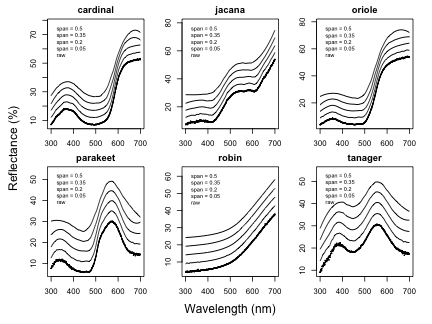From the resulting plot, we can see that span = 0.2 is the minimum amount of smoothing to remove spectral noise while preserving the original spectral shape. Based on this value, we will now use the opt argument in procspec to smooth data for further plotting and analysis. spec.sm <- procspec(sppspec, opt = "smooth", span = 0.2)  ## processing options applied: ## smoothing spectra with a span of 0.2  plot(sppspec[, 5] ~ sppspec[, 1], type = "l", lwd = 10, col = "grey", xlab = "Wavelength (nm)", ylab = "Reflectance (%)" ) lines(spec.sm[, 5] ~ sppspec[, 1], col = "red", lwd = 2)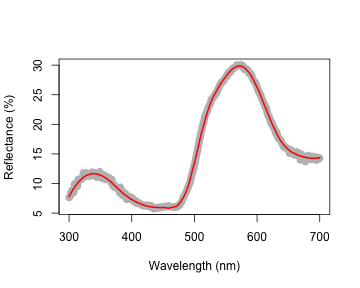We can also try different normalizations. Options include subtracting the minimum reflectance of a spectrum at all wavelengths (effectively making the minimum reflectance equal to zero, opt = "min", left panel, below) and making the reflectance at all wavelength proportional to the maximum reflectance (i.e. setting maximum reflectance to 1; opt = "max", center panel, below). Note that the user can specify multiple processing options that will be applied sequentially to the spectral data by procspec (right panel, below). # Run some different normalizations specs.max <- procspec(sppspec, opt = "max")  ## processing options applied: ## Scaling spectra to a maximum value of 1  specs.min <- procspec(sppspec, opt = "min")  ## processing options applied: ## Scaling spectra to a minimum value of zero  specs.str <- procspec(sppspec, opt = c("min", "max")) # multiple options  ## processing options applied: ## Scaling spectra to a minimum value of zero ## Scaling spectra to a maximum value of 1  # Plot results par(mfrow = c(1, 3), mar = c(2, 2, 2, 2), oma = c(3, 3, 0, 0)) plot(specs.min[, 5] ~ c(300:700), xlab = "", ylab = "", type = "l") abline(h = 0, lty = 2) plot(specs.max[, 5] ~ c(300:700), ylim = c(0, 1), xlab = "", ylab = "", type = "l") abline(h = c(0, 1), lty = 2) plot(specs.str[, 5] ~ c(300:700), type = "l", xlab = "", ylab = "") abline(h = c(0, 1), lty = 2) mtext("Wavelength (nm)", side = 1, outer = TRUE, line = 1) mtext("Reflectance (%)", side = 2, outer = TRUE, line = 1)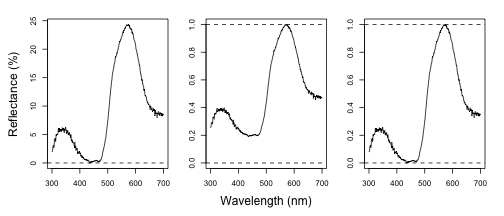## Binning and PCA Analysis of Spectral Shape Another intended usage of procspec is preparation of spectral data for variable reduction (for example, using Principal Component Analysis, or PCA). Following Cuthill et al. (1999), we can use opt = 'center' to center spectra to have a mean reflectance of zero (thus removing brightness as a dominant variable in the PCA) and then bin the spectra into user-defined bins (using the opt = 'bin' argument) to obtain a dataframe suitable for the PCA. # pca analysis spec.bin <- procspec(sppspec, opt = c("bin", "center"))  ## processing options applied: ## Centering spectra to a mean of zero ## binned spectra to 21-nm intervals  head(spec.bin) spec.bin <- t(spec.bin) # transpose so wavelength are variables for the PCA colnames(spec.bin) <- spec.bin[1, ] # names variables as wavelength bins spec.bin <- spec.bin[-1, ] # remove 'wl' column pca1 <- prcomp(spec.bin, scale = TRUE)  summary(pca1)  ## Importance of components: ## PC1 PC2 PC3 PC4 PC5 PC6 ## Standard deviation 3.6041 1.9853 1.5800 0.66314 0.36427 2.705e-16 ## Proportion of Variance 0.6495 0.1971 0.1248 0.02199 0.00663 0.000e+00 ## Cumulative Proportion 0.6495 0.8466 0.9714 0.99337 1.00000 1.000e+00  As can be seen by the summary, PC1 explains approximately 64% of the variation in spectral shape and describes the relative amount of long wavelengths reflected. The flexibility of R and pavo's plotting capabilities allows you to sort spectra by another variable (e.g., PC1 loading) and then plot in a stacked format using the plot function. # Generate colors from spectra colr <- spec2rgb(sppspec) wls <- as.numeric(colnames(spec.bin)) # Rank specs by PC1 sel <- rank(pca1$x[, 1])
sel <- match(names(sort(sel)), names(sppspec))

# Plot results
par(mfrow = c(1, 2), mar = c(2, 4, 2, 2), oma = c(2, 0, 0, 0))
abline(h = 0, lty = 2)
plot(sppspec, select = sel, type = "s", col = spec2rgb(sppspec))
mtext("Wavelength (nm)", side = 1, outer = TRUE, line = 1)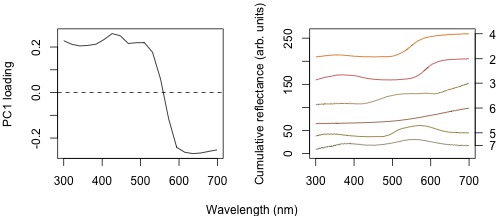## Dealing With Negative Values in Spectra

Negative values in spectra are unwanted, as they are uninterpretable (how can there be less than zero light reflected by a surface?) and can affect estimates of color variables. Nonetheless, certain spectrometer manufacturers allow negative values to be saved. To handle negative values, the procspec function has an argument called fixneg. The two options available are (1) adding the absolute value of the most negative value to the whole spectrum with addmin, and (2) changing all negative values to zero with zero.

# Create a duplicate spectrum and add some negative values
refl <- sppspec[, 7] - 20
testspecs <- as.rspec(cbind(c(300:700), refl))

## wavelengths found in column 1

# Apply two different processing options
testspecs.fix1 <- procspec(testspecs, fixneg = "addmin")

## processing options applied:
## Negative value correction: added min to all reflectance

testspecs.fix2 <- procspec(testspecs, fixneg = "zero")

## processing options applied:
## Negative value correction: converted negative values to zero

# Plot it
par(mar = c(2, 2, 2, 2), oma = c(3, 3, 0, 0))
layout(cbind(c(1, 1), c(2, 3)), widths = c(2, 1, 1))

plot(testspecs, select = 2, ylim = c(-10, 30))
abline(h = 0, lty = 3)

plot(testspecs.fix1, select = 2, ylim = c(-10, 30))
abline(h = 0, lty = 3)

plot(testspecs.fix2, select = 2, ylim = c(-10, 30))
abline(h = 0, lty = 3)

mtext("Wavelength (nm)", side = 1, outer = TRUE, line = 1)
mtext("Reflectance (%)", side = 2, outer = TRUE, line = 1)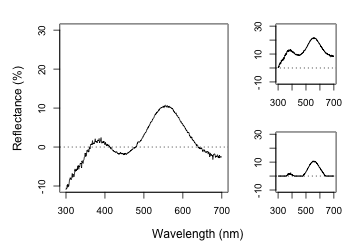These manipultions may have different effects on the final spectra, which the user should keep in mind and use according to the final goal of the analysis. For example, by adding the minimum reflectance to all other wavelength, the shape of the curve is preserved, but the maximum reflectance is much higher. On the other hand, substituting negative values with zero preserves absolute reflectance values, but may cause the spectral shape to be lost. The "best” transformation will depend on the severity of the problem of negative values and the goal of the analysis (e.g. will reflectance intensity be used? What is more important, to preserve reflectance values or the total shape of the curve?). Which correction to use would also depend on the source of the negative values. If they are thought to originate from improper calibration of the spectrophotometer, fixneg = addmin would be appropriate. However, if they are thought to originate from electric noise, fixneg = zero would be more appropriate.

# Visualizing Spectral Data

pavo offers three main plotting functions. The main one is plot, which combines several different options in a flexible framework for most commonly used purposes. The explorespec function aims at providing initial exploratory analysis, as demonstrated in Section 1. Finally, aggplot provides a simple framework for publication-quality plots of aggregated spectral data.

## The plot Function Options

Since pavo uses the class rspec to identify spectral data, the function plot.rspec can be called simply by calling plot(data). If the object is not of class rspec the multivariate visualization methods will not work as expected, so it might be useful to check the data using is.rspec and convert with as.rspec if necessary.

We have implemented three methods of visualizing spectral data using plot:

1. Overlay: all spectra plotted with same x- and y-axis
2. Stack: spectra plotted with same x-axis but arranged vertically along y-axis
3. Heatmap: false color map to illustrate three dimensional data

These options are in addition to the exploratory plotting offered by explorespec, as seen in the figures in section 1. To showcase the capabilities of plot.rspec, we will use the teal dataset included in pavo. This dataset consists of reflectance spectra from the iridescent wing patch of a green-winged teal (Anas carolinensis). Reflectance measurements were taken between 300 and 700 nm at different incident angles, ranging from 15o^ to 70o^ (in 5o^ increments) (Eliason & Shawkey, 2012).

### The overlay Option

We can start out by visualizing these spectra with the overlay option in plot. Another neat option pavo offers is to convert reflectance spectra to their approximate perceived color, by using the function spec2rgb. This can make for some very interesting plots and even exploratory data analysis, as shown above.

par(mar = c(4, 4, 2, 2))
data(teal)
plot(teal, type = "o", col = spec2rgb(teal))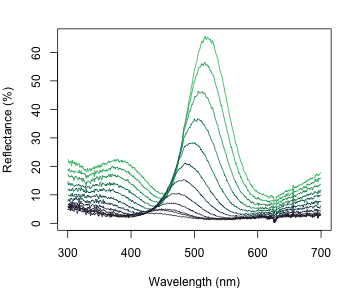### The stack Option

Another option is the stack plot (again, with human vision approximations of the color produced by the spectra using spec2rgb).

teal.norm <- procspec(teal, opt = c("min", "max"))

## processing options applied:
## Scaling spectra to a minimum value of zero
## Scaling spectra to a maximum value of 1

par(mfrow = c(1, 2), mar = c(2, 2, 2, 2), oma = c(2, 2, 0, 0))

plot(teal, type = "s", col = spec2rgb(teal))
plot(teal.norm, type = "s", col = spec2rgb(teal))

mtext("Wavelength (nm)", side = 1, outer = T, line = 1)
mtext("Cumulative reflectance (A.U.)", side = 2, outer = T, line = 1)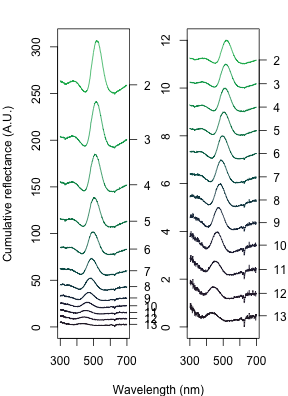Note that in the above figure, the y axis to the right includes the index of each spectrum. This makes it easier to identify and subset specific spectra or groups of spectra using the select argument in plot.rspec. Note also that the first index is actually 2, preserving the sequence in the original dataset (since the first column is wavelength). Though this may seem confusing at first (“why is my first spec number 2?”“) this preserves subsetting hierarchy: using plot(teal, select = 2) will show the same spectra that would be selected if you use teal[ ,2].

### The heatmap Option

Since this dataset is three-dimensional (containing wavelengths, reflectance values and incident angles) we can also use the heatmap function. First, it will be necessary to define a vector for the incident angles each spectrum was measured at:

angles <- seq(15, 70, by = 5)


Next, we will smooth the data with procspec and plot as a false color map (heatmap):

teal.sm <- procspec(teal, opt = c("smooth"))

## processing options applied:
## smoothing spectra with a span of 0.25

plot(teal.sm,
type = "h", varying = angles,
ylab = expression(paste("Incident angle (", degree, ")")),
las = 1, useRaster = TRUE
)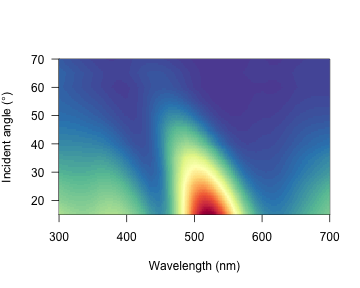These plots can be very useful to observe changes over time, for example, or any other type of continuous variation.

## The aggplot Function

aggplot has a very similar interface to aggspec, allowing for quick plotting of aggregated spectra combined by a factor, such as species, sex, experimental treatment, and so on. Its main output is a plot with lines of group mean spectra outlined by a shaded area indicating some measure of variability, such as the standard deviation of the group. Note that functions that aren't already implemented in R must be passed like they would be to functions such as apply (e.g., function(x)sd(x)/sqrt(length(x)) in the example below).

par(mfrow = c(1, 2), mar = c(4, 4, 2, 2), oma = c(2, 0, 0, 0))

# Plot using median and standard deviation, default colors
aggplot(mspecs, spp, FUN.center = median, alpha = 0.3, legend = TRUE)

# Plot using mean and standard error, in greyscale
aggplot(mspecs, spp,
FUN.error = function(x) sd(x) / sqrt(length(x)),
lcol = 1, shadecol = "grey", alpha = 0.7
)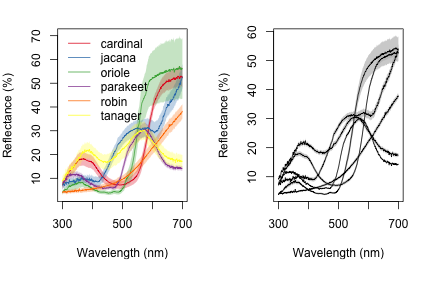# Analyzing Spectral Data

## Overview

pavo offers two main approaches for spectral data analysis. First, color variables can be calculated based on the shape of the reflectance spectra. By using special R classes for spectra data frame objects, this can easily be done using the summary function with an rspec object (see below). The function peakshape also returns descriptors for individual peaks in spectral curves, as outlined below.

Second, reflectance spectra can be analyzed by accounting for the visual system receiving the color signal, therefore representing reflectance spectra as perceived colors. To that end, we have implemented a suite of visual models and colorspaces including; the receptor-noise limited model of model of Vorobyev & Osorio (1998), Endler's (1990) segment classification method, flexible di- tri- and tetra-chromatic colorspaces, the Hexagon model of Chittka (1992), the color-opponent coding space of Backhaus (1991), CIELAB and CIEXYZ spaces, and the categorical model of Troje (1992).

## Spectral Shape Analysis

### Colorimetric Variables

Obtaining colorimetric variables (peratining to hue, saturation and brightness/value) is pretty straightforward in pavo. Since reflectance spectra is stored in an object of class rspec, the summary function recognizes the object as such and extracts 23 variables, as outlined in Montgomerie (2006). Though outlined in a book chapter on bird coloration, these variables are broadly applicable to any reflectance data, particularly if the taxon of interest has color vision within the UV-human visible range.

The description and formulas for these variables can be found by running ?summary.rspec.

summary(spec.sm)


summary also takes an additional argument subset which if changed from the default FALSE to TRUE will return only the most commonly used colorimetrics (Andersson 2006). summary can also take a vector of color variable names, which can be used to filter the results

summary(spec.sm, subset = TRUE)

# Extract only brightness variables
summary(spec.sm, subset = c('B1', 'B2', 'B3'))


### Peak Shape Descriptors

Particularly in cases of reflectance spectra that have multiple discrete peaks (in which case the summary function will only return variables based on the tallest peak in the curve), it might be useful to obtain variables that describe individual peak's properties. The peakshape function identifies the peak location (H1), returns the reflectance at that point (B3), and identifies the wavelengths at which the reflectance is half that at the peak, calculating the wavelength bandwidth of that interval (the Full Width at Half Maximum, or FWHM). The function also returns the half widths, which are useful when the peaks are located near the edge of the measurement limit and half maximum reflectance can only be reliably estimated from one of its sides.

If this all sounds too esoteric, fear not: peakshape has the option of returning plots indicating what it's calculating. The vertical continuous red line indicates the peak location, the horizontal continuous red line indicates the half-maximum reflectance, and the distance between the dashed lines (HWHM.l and HWHM.r) is the FWHM:

par(mfrow = c(2, 3))
peakshape(spec.sm, plot = TRUE)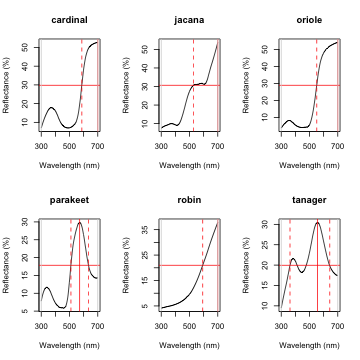##         id       B3  H1 FWHM HWHM.l HWHM.r incl.min
## 1 cardinal 52.70167 700   NA    113     NA      Yes
## 2   jacana 53.78744 700   NA    171     NA      Yes
## 3   oriole 54.15508 700   NA    149     NA      Yes
## 4 parakeet 29.86504 572  125     62     63      Yes
## 5    robin 37.85542 700   NA    107     NA      Yes
## 6  tanager 30.48108 557  281    195     86      Yes


As it can be seen, the variable FWHM is meaningless if the curve doesn't have a clear peak. Sometimes, such as in the case of the Cardinal (Above figure, first panel), there might be a peak which is not the point of maximum reflectance of the entire spectral curve. The half-width can also be erroneously calculated when there are two peaks, as can be seen in the case of the Tanager (Above figure, last panel). In this case, it's useful to set wavelength limits when calculating the FWHM by using the lim argument. peakshape also offers a select argument to facilitate subsetting the spectra data frame to, for example, focus on a single reflectance peak:

peakshape(spec.sm, select = 2, lim = c(300, 500), plot = TRUE)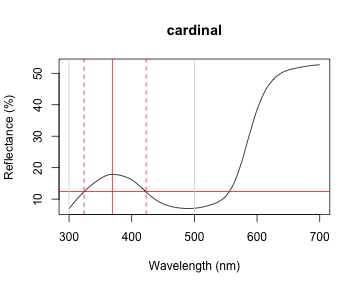##         id       B3  H1 FWHM HWHM.l HWHM.r incl.min
## 1 cardinal 17.84381 369   99     45     54      Yes


## Visual Modelling

### Overview

As of version 1.0, all visual and colorspace modelling and plotting options are wrapped into four main function: vismodel, colspace, coldist, and plot. As detailed below, these functions cover the basic processes common to most modelling approaches. Namely, the estimation of quantum catches, their conversion to an appropriate space, estimating the distances between samples, and visualising the results. As a brief example, a typical workflow for modelling might use:

1. vismodel to estimate photoreceptor quantum catches. The assumptions of visual models may differ dramatically, so be sure to select options that are appropriate for your intended use.
2. colspace to convert the results of vismodel (or user-provided quantum catches) into points in a given colorspace, specified with the space argument. If no space argument is provided, colspace will automatically select the di-, tri- or tetrahedral colorspace, depending on the nature of the input data. The result of colspace will be an object of class colspace (that inherits from data.frame), and will contain the location of stimuli in the selected space along with any associated color variables.
3. coldist to estimate the color distances between points in a colourspace (if the input is from colspace), or the 'perceptual', noise-weighted distances in the receptor-noise limited model (if the input is from vismodel where relative = FALSE).
4. plot the output. plot will automatically select the appropriate visualisation based on the input colspace object, and will also accept various graphical parameters depending on the colorspace (see ?plot.colspace and links therein for details).

### Accessing and Plotting In-built Spectral Data

All in-built spectral data in pavo can be easily retrieved and/or plotted via the sensdata() function. These data can be visualised directly by including plot = TRUE, or assigned to an object for subsequent use, as in:

musca_sense <- sensdata(visual = "musca", achromatic = "md.r1")

##    wl    musca.u     musca.s     musca.m     musca.l       md.r1
## 1 300 0.01148992 0.006726680 0.001533875 0.001524705 0.001713692
## 2 301 0.01152860 0.006749516 0.001535080 0.001534918 0.001731120
## 3 302 0.01156790 0.006773283 0.001536451 0.001545578 0.001751792
## 4 303 0.01160839 0.006798036 0.001537982 0.001556695 0.001775960
## 5 304 0.01165060 0.006823827 0.001539667 0.001568283 0.001803871
## 6 305 0.01169510 0.006850712 0.001541499 0.001580352 0.001835777


### Visual Phenotypes

pavo contains numerous di-, tri- and tetrachromatic visual systems, to accompany the suite of new models. The full complement of included systems are accessible via the vismodel() argument visual: : avg.uv average ultraviolet-sensitive avian (tetrachromat) : avg.v average violet-sensitive avian (tetrachromat) : bluetit The blue tit Cyanistes caeruleus (tetrachromat) : star The starling Sturnus vulgaris (tetrachromat) : pfowl The peafowl Pavo cristatus (tetrachromat) : apis The honeybee Apis mellifera (trichromat) : ctenophorus The ornate dragon lizard Ctenophorus ornatus (trichromat) : canis The canid Canis familiaris (dichromat) : musca The housefly Musca domestica (tetrachromat) : cie2 2-degree color matching functions for CIE models of human color vision (trichromat) : cie10 10-degree color matching functions for CIE models of human color vision (trichromat) : segment A generic 'viewer' with broad sensitivities for use in the segment analysis of Endler (1990) (tetrachromat) : habronattus The jumping spider Habronattus pyrrithrix (trichromat). : rhinecanthus The triggerfish Rhinecanthus aculeatus (trichromat).

### Estimating Quantum Catch

Numerous models have been developed to understand how colors are perceived and discriminated by an individual's or species' visual system (Described in detail in Endler 1990; Renoult et al. 2015; Vorobyev 1998). In essence, these models take into account the receptor sensitivity of the different receptors that make the visual system in question and quantify how a given color would stimulate those receptors individually, and their combined effect on the perception of color. These models also have an important component of assuming and interpreting the chromatic component of color (hue and saturation) to be processed independently of the achromatic (brightness, or luminance) component. This provides a flexible framework allowing for a tiered model construction, in which information on aspects such as different illumination sources, backgrounds, and visual systems can be considered and compared.

To apply any such model, we first need to quantify receptor excitation and then consider how the signal is being processed, while possibly considering the relative density of different cones and the noise-to-signal ratio.

To quantify the stimulation of cones by the emitted color, we will use the pavo function vismodel. This function takes an rspec dataframe as a minimal input, and the user can either select from the available options or input its own data for the additional arguments in the function:

• visual: the visual system to be used. Available inbuilt options are detailed above, or the user may include their own dataframe, with the first column being the wavelength range and the following columns being the absorbance at each wavelength for each cone type (see below for an example).
• achromatic: Either a receptor's sensitivity data (available options include the blue tit, chicken, and starling double-cones, and the housefly's R1-6 receptor), which can also be user-defined as above; or the longest-wavelength receptor, the sum of the two longest-wavelength receptors, or the sum of all receptors can be used. Alternatively, none can be specified for no achromatic stimulation calculation.
• illum: The illuminant being considered. By default, it considers an ideal white illuminant, but implemented options are a blue sky, standard daylight, and forest shade illuminants. A vector of same length as the wavelength range being considered can also be used as the input.
• trans: Models of the effects of light transmission (e.g. through noisy environments or ocular filters). The argument defaults to ideal (i.e. no effect), though users can also use the built-in options of bluetit or blackbird to model the ocular transmission of blue tits/blackbirds, or specify a user-defined vector containing transmission spectra.
• qcatch: This argument determines what photon catch data should be returned
• Qi: The receptor quantum catches, calculated for receptor $$i$$ as: $Q_i = \int_\lambda{R_i(\lambda)S(\lambda)I(\lambda)d\lambda}$ Where $$\lambda$$ denotes the wavelength, $$R_i(\lambda)$$ the spectral sensitivity of receptor $$i$$, $$S (\lambda)$$ the reflectance spectrum of the color, and $$I(\lambda)$$ the illuminant spectrum.
• fi: The receptor quantum catches transformed according to Fechner's law, in which the signal of the receptor is proportional to the logarithm of the quantum catch i.e. $$f_i = ln(Q_i)$$
• Ei: the hyperbolic transform (a simplification of the Michaelis–Menton photoreceptor equation), where $E_i = \frac{Q_i}{Q_i + 1}$.
• bkg: The background being considered. By default, it considers an idealized background (i.e. wavelength-independent influence of the background on color). A vector of same length as the wavelength range being considered can also be used as the input.
• vonkries: a logical argument which determines if the von Kries transformation (which normalizes receptor quantum catches to the background, thus accounting for receptor adaptation) is to be applied (defaults to FALSE). If TRUE, $$Q_i$$ is multiplied by a constant $$k$$, which describes the von Kries transformation: $k_i = \frac{1} { \int_\lambda{R_i(\lambda)S^b(\lambda)I(\lambda)d\lambda }, }$ Where $$S^b$$ denotes the reflectance spectra of the background.
• scale: This argument defines how the illuminant should be scaled. The scale of the illuminant is critical of receptor noise models in which the signal intensity influences the noise (see Receptor noise section, below). Illuminant curves should be in units of $$\mu mol.s^{-1}.m^{-2}$$ in order to yield physiologically meaningful results. (Some software return illuminant information values in $$\mu Watt.cm^{-2}$$, and must be converted to $$\mu mol.s^{-1}.m^{-2}$$. This can be done by using the irrad2flux and flux2irrad functions.) Therefore, if the user-specified illuminant curves are not in these units (i.e. are measured proportional to a white standard, for example), the scale parameter can be used as a multiplier to yield curves that are at least a reasonable approximation of the illuminant value. Commonly used values are 500 for dim conditions and 10,000 for bright conditions.
• relative: If TRUE, it will make the cone stimulations relative to their sum. This is appropriate for colorspace models such as the avian tetrahedral colorspace (Goldsmith 1990, Stoddard 2008; For the photon catch and neural noise model, it is important to set} relative = FALSE).

All visual models begin with the estimation of receptor quantum catches. The requirements of models may differ significantly of course, so be sure to consult the function documentation and original publications. For this example, we will use the average reflectance of the different species to calculate the raw stimulation of retinal cones, considering the avian average UV visual system, a standard daylight illumination, and an idealized background.

vismod1 <- vismodel(sppspec,
visual = "avg.uv", achromatic = "bt.dc",
illum = "D65", relative = FALSE
)
vismod1


Since there are multiple parameters that can be used to customize the output of vismodel, as detailed above, for convenience these can be returned by using summary in a vismodel object:

summary(vismod1)

## visual model options:
##  * Quantal catch: Qi
##  * Visual system, chromatic: avg.uv
##  * Visual system, achromatic: bt.dc
##  * Illuminant: D65, scale = 1 (von Kries color correction not applied)
##  * Background: ideal
##  * Transmission: ideal
##  * Relative: FALSE

##        u                 s                 m                l
##  Min.   :0.01093   Min.   :0.03749   Min.   :0.1297   Min.   :0.2171
##  1st Qu.:0.01509   1st Qu.:0.06137   1st Qu.:0.1695   1st Qu.:0.2318
##  Median :0.01925   Median :0.06353   Median :0.2595   Median :0.2866
##  Mean   :0.02321   Mean   :0.08854   Mean   :0.2262   Mean   :0.3197
##  3rd Qu.:0.03059   3rd Qu.:0.12753   3rd Qu.:0.2722   3rd Qu.:0.3969
##  Max.   :0.04177   Max.   :0.15716   Max.   :0.2923   Max.   :0.4807
##       lum
##  Min.   :0.1501
##  1st Qu.:0.1965
##  Median :0.2194
##  Mean   :0.2215
##  3rd Qu.:0.2618
##  Max.   :0.2755


We can visualize what vismodel is doing when estimating quantum catches by comparing the reflectance spectra to the estimates they are generating:

par(mfrow = c(2, 6), oma = c(3, 3, 0, 0))
layout(rbind(c(2, 1, 4, 3, 6, 5), c(1, 1, 3, 3, 5, 5), c(8, 7, 10, 9, 12, 11), c(7, 7, 9, 9, 11, 11)))

sppspecol <- as.character(spec2rgb(sppspec))

for (i in 1:6) {
par(mar = c(2, 2, 2, 2))
plot(sppspec, select = i + 1, col = sppspecol[i], lwd = 3, ylim = c(0, 100))
par(mar = c(4.1, 2.5, 2.5, 2))
barplot(as.matrix(vismod1[i, 1:4]), yaxt = "n", col = "black")
}

mtext("Wavelength (nm)", side = 1, outer = TRUE, line = 1)
mtext("Reflectance (%)", side = 2, outer = TRUE, line = 1)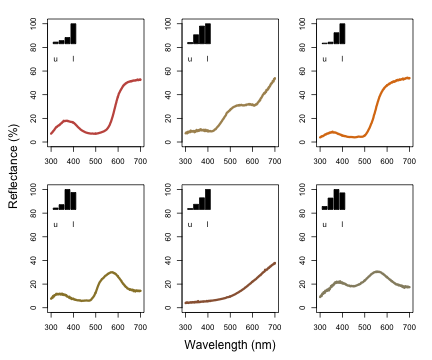As described above, vismodel also accepts user-defined visual systems, background and illuminants. We will illustrate this by showcasing the function sensmodel, which models spectral sensitivities of retinas based on their peak cone sensitivity, as described in Govardovskii et al. (2000) and Hart & Vorobyev (2005). sensmodel takes several optional arguments, but the main one is a vector containing the peak sensitivities for the cones being modeled. Let's model an idealized dichromat visual system, with cones peaking in sensitivity at 350 and 650 nm:

idealizeddichromat <- sensmodel(c(350, 650))
plot(idealizeddichromat, col = spec2rgb(idealizeddichromat), ylab = "Absorbance")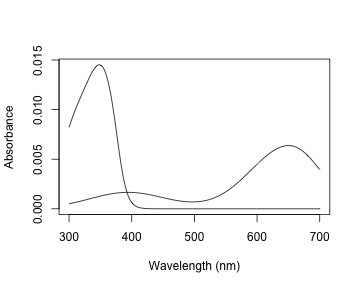vismod.idi <- vismodel(sppspec, visual = idealizeddichromat, relative = FALSE)
vismod.idi


### The Receptor Noise Model

The receptor-noise limited model of Vorobyev (1998, 2001) offers a basis for estimating the 'perceptual' distance between coloured stimuli (depending on available physiological and behavioural data), and assumes that the simultaneous discrimination of colors is fundamentally limited by photoreceptor noise. Color distances under the RNL model can be calculated by using the inverse of the noise-to-signal ratio, known as the Weber fraction ($$w_i$$ for each cone $$i$$). The Weber fraction can be calculated from the noise-to-signal ratio of cone $$i$$ ($$v_i$$) and the relative number of receptor cells of type $$i$$ within the receptor field ($$n_i$$):

$w_i = \frac{v_i}{\sqrt{n_i}}$

$$w_i$$ is the value used for the noise when considering only neural noise mechanisms. Alternatively, the model can consider that the intensity of the color signal itself contributes to the noise (photoreceptor, or quantum, noise). In this case, the noise for a receptor $$i$$ is calculated as:

$w_i = \sqrt{\frac{v_i^2}{\sqrt{n_i}} + \frac{2}{Q_a+Q_b}}$

where $$a$$ and $$b$$ refer to the two color signals being compared. Note that when the values of $$Q_a$$ and $$Q_b$$ are very high, the second portion of the equation tends to zero, and the both formulas should yield similar results. Hence, it is important that the quantum catch are calculated in the appropriate illuminant scale, as described above.

Color distances are obtained by weighting the Euclidean distance of the photoreceptor quantum catches by the Weber fraction of the cones ($$\Delta S$$). These measurements are in units of Just Noticeable Differences (JNDs), where distances over a certain threshold (usually 1) are considered to be discernible under the conditions considered (e.g., backgrounds, illumination). The equations used in these calculations are:

For dichromats: $\Delta S = \sqrt{\frac{(\Delta f_1 - \Delta f_2)^2}{w_1^2+w_2^2}}$

For trichromats: $\Delta S = \sqrt{\frac{w_1^2(\Delta f_3 - \Delta f_2)^2 + w_2^2(\Delta f_3 - \Delta f_1)^2 + w_3^2(\Delta f_1 - \Delta f_2)^2 }{ (w_1w_2)^2 + (w_1w_3)^2 + (w_2w_3)^2 }}$

For tetrachromats: $\Delta S = \sqrt{(w_1w_2)^2(\Delta f_4 - \Delta f_3)^2 + (w_1w_3)^2(\Delta f_4 - \Delta f_2)^2 + (w_1w_4)^2(\Delta f_3 - \Delta f_2)^2 + \ (w_2w_3)^2(\Delta f_4 - \Delta f_1)^2 + (w_2w_4)^2(\Delta f_3 - \Delta f_1)^2 + (w_3w_4)^2(\Delta f_2 - \Delta f_1)^2 / \ ((w_1w_2w_3)^2 + (w_1w_2w_4)^2 + (w_1w_3w_4)^2 + (w_2w_3w_4)^2)}$

For the chromatic contrast. The achromatic contrast ($$\Delta L$$) can be calculated based on the double cone or the receptor (or combination of receptors) responsible for chromatic processing by the equation:

$\Delta L = \frac{\Delta f}{w}$

#### Perceptual Distances with coldist

pavo implements the noise-weighted color distance calculations in the function coldist, assuming that raw receptor quantum catches are provided (via relative = FALSE in vismodel()). For the achromatic contrast, coldist uses n4 to calculate $$w$$ for the achromatic contrast. Note that even if $$Q_i$$ is chosen, values are still log-transformed. This option is available in case the user wants to specify a data frame of quantum catches that was not generated by vismodel as an input. In this case, the argument qcatch should be used to inform the function if $$Q_i$$ or $$f_i$$ values are being used (note that if the imput to coldist is an object generated using the vismodel function, this argument is ignored.) The type of noise to be calculated can be selected from the coldist argument noise (which accepts either "neural" or "quantum").

coldist(vismod1,
noise = "neural", achro = TRUE, n = c(1, 2, 2, 4),
weber = 0.1, weber.achro = 0.1
)

##      patch1   patch2        dS        dL
## 1  cardinal   jacana  8.423965 3.3449627
## 2  cardinal   oriole  7.905876 3.5110820
## 3  cardinal parakeet  7.999730 0.5177495
## 4  cardinal    robin  5.805562 2.5606167
## 5  cardinal  tanager 10.100311 1.9068807
## 6    jacana   oriole 10.151253 0.1661193
## 7    jacana parakeet  4.231570 2.8272132
## 8    jacana    robin  3.503769 5.9055794
## 9    jacana  tanager  5.126266 1.4380820
## 10   oriole parakeet  8.135793 2.9933325
## 11   oriole    robin  7.220505 6.0716987
## 12   oriole  tanager 13.608253 1.6042013
## 13 parakeet    robin  4.606847 3.0783661
## 14 parakeet  tanager  5.969760 1.3891312
## 15    robin  tanager  7.688707 4.4674974

coldist(vismod.idi, n = c(1, 2), weber = 0.1)

##      patch1   patch2        dS
## 1  cardinal   jacana 2.0993283
## 2  cardinal   oriole 4.6567462
## 3  cardinal parakeet 2.2575457
## 4  cardinal    robin 3.7236780
## 5  cardinal  tanager 3.5417700
## 6    jacana   oriole 2.5574178
## 7    jacana parakeet 4.3568740
## 8    jacana    robin 1.6243496
## 9    jacana  tanager 5.6410983
## 10   oriole parakeet 6.9142918
## 11   oriole    robin 0.9330682
## 12   oriole  tanager 8.1985162
## 13 parakeet    robin 5.9812236
## 14 parakeet  tanager 1.2842244
## 15    robin  tanager 7.2654480


Where dS is the chromatic contrast ($$\Delta S$$) and dL is the achromatic contrast ($$\Delta L$$). Note that, by default, achro = FALSE, so dL isn't included in the second result (this is to maintain consistency since, in the vismodel function, the achromatic argument defaults to none). As expected, values are really high under the avian color vision, since the colors of these species are quite different and because of the enhanced discriminatory ability with four compared to two cones.

coldist also has a subset argument, which is useful if only certain comparisons are of interest (for example, of color patches against a background, or only comparisons among a species or body patch). subset can be a vector of length one or two. If only one subsetting option is passed, all comparisons against the matching argument are returned (useful in the case of comparing to a background, for example). If two values are passed, comparisons will only be made between samples that match that rule (partial string matching and regular expressions are accepted). For example, compare:

coldist(vismod1, subset = 'cardinal')


to:

coldist(vismod1, subset = c('cardinal', 'jacana'))


#### Converting receptor noise-corrected distances to Cartesian coordinates

You can convert distances in JND back to Cartesian position coordinates with the function jnd2xyz. Note that the actual position of these points in the XYZ space is arbitrary; therefore the function allows you to rotate the data so that, for example, the vector leading to the long-wavelength cone aligns with the X axis:

fakedata1 <- sapply(
seq(100, 500, by = 20),
function(x) rowSums(cbind(
dnorm(300:700, x, 30),
dnorm(300:700, x + 400, 30)
))
)

# Creating idealized specs with varying saturation
fakedata2 <- sapply(
c(500, 300, 150, 105, 75, 55, 40, 30),
function(x) dnorm(300:700, 550, x)
)

fakedata1 <- as.rspec(data.frame(wl = 300:700, fakedata1))

## wavelengths found in column 1

fakedata1 <- procspec(fakedata1, "max")

## processing options applied:
## Scaling spectra to a maximum value of 1

fakedata2 <- as.rspec(data.frame(wl = 300:700, fakedata2))

## wavelengths found in column 1

fakedata2 <- procspec(fakedata2, "sum")

## processing options applied:
## Scaling spectra to a total area of 1

fakedata2 <- procspec(fakedata2, "min")

## processing options applied:
## Scaling spectra to a minimum value of zero

# Converting reflectance to percentage
fakedata1[, -1] <- fakedata1[, -1] * 100
fakedata2[, -1] <- fakedata2[, -1] / max(fakedata2[, -1]) * 100

# Combining and converting to rspec
fakedata.c <- data.frame(wl = 300:700, fakedata1[, -1], fakedata2[, -1])
fakedata.c <- as.rspec(fakedata.c)

## wavelengths found in column 1

# Visual model and color distances
fakedata.vm <- vismodel(fakedata.c, relative = FALSE, achro = TRUE)
fakedata.cd <- coldist(fakedata.vm,
noise = "neural", n = c(1, 2, 2, 4),
weber = 0.1, achro = TRUE
)

# Converting to Cartesian coordinates
fakedata.cc <- jnd2xyz(fakedata.cd, ref1 = "l", axis1 = c(1, 0, 0), ref2 = NULL)

##             x          y         z      lum
## X1 -26.165302   3.692783 -17.71718 1.533258
## X2 -11.212399  -2.085407 -27.97937 1.533258
## X3   3.076291  -7.441594 -37.76761 1.533258
## X4  16.523287 -12.475488 -46.33606 1.533255
## X5  25.812150 -17.848152 -40.71621 1.533213
## X6  31.912643 -23.368560 -24.79660 1.532744


which you can then plot as well:

plot(fakedata.cc, theta = 55, phi = 25, col = spec2rgb(fakedata.c))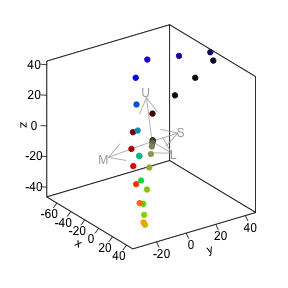The axes in this colorspace are in JND units. For more information on these functions, see ?jnd2xyz, ?jndrot and ?jndplot.

### Colorspaces

Another general, flexible representation of stimuli is the color space model. In such approaches, photon catches are expressed in relative values (so that the quantum catches of all receptors involved in chromatic discrimination sum to 1). The maximum stimulation of each cone $$n$$ is placed at the vertex of a $$(n-1)$$-dimensional polygon that encompasses all theoretical colors that can be perceived by that visual system. For the avian visual system comprised of 4 cones, for example, all colors can be placed somewhere in the volume of a tetrahedron, in which each of the four vertices represents the maximum stimulation of that particular cone type.

Though these models do not account for receptor noise (and thus do not allow an estimate of JNDs), they presents several advantages. First, they make for a very intuitive representation of color points accounting for attributes of the color vision of the signal receiver. Second, they allow for the calculation of several interesting variables that represent color. For example, hue can be estimated from the angle of the point relative to the xy plane (blue-green-red) and the z axis (UV); saturation can be estimated as the distance of the point from the achromatic center.

data(flowers) is a new example dataset consisting of reflectance spectra from 36 Australian angiosperm species, which we'll use to illustrate for the following colorspace modelling.

data(flowers)


##    wl Goodenia_heterophylla Goodenia_geniculata Goodenia_gracilis
## 1 300             1.7426387            1.918962         0.3638354
## 2 301             1.5724849            1.872966         0.3501921
## 3 302             1.4099808            1.828019         0.3366520
## 4 303             1.2547709            1.784152         0.3231911
## 5 304             1.1064997            1.741398         0.3097854
## 6 305             0.9648117            1.699792         0.2964107


#### Di-, Tri-, and Tetrachromatic Colorspaces

As of version 1.0, pavo has expanded modeling and visualization capabilities for generic di- and trichromatic spaces, uniting these approaches in a cohesive workflow. As with most colorspace models, we first estimate relative quantum catches with various assumptions by using the vismodel function, before converting each set of values to a location in colorspace by using the space argument in colspace (the function can also be set to try to detect the dimensionality of the colorspace automatically). For di- tri- and tetrachromatic spaces, colspace calculates the coordinates of stimuli as:

Dichromats: $x = \frac{1}{\sqrt{2}}(Q_l - Q_s)$

Trichromats: $x = \frac{1}{\sqrt{2}}(Q_l - Q_m)$ $y = \frac{\sqrt{2}}{\sqrt{3}}(Q_s - \frac{Q_l + Q_m}{2})$

Tetrachromats: $x = \frac{1}{\sqrt{2}}(Q_l - Q_m)$ $y = \frac{\sqrt{2}}{\sqrt{3}}(Q_s - \frac{Q_l + Q_m}{2})$ $z = \frac{\sqrt{3}}{2}(Q_u - \frac{Q_l + Q_m + Q_s}{3})$

Where Q~u~, Q~s~, Q~m~, and Q~l~ refer to quantum catch estimates for UV-, short, medium-, and long-wavelength photoreceptors, respectively.

For a dichromatic example, we can model our floral reflectance data using the visual system of the domestic dog Canis familiaris, which has two cones with maximal sensitivity near 440 and 560 nm.

vis.flowers <- vismodel(flowers, visual = 'canis')

di.flowers <- colspace(vis.flowers, space = 'di')


##                                s         l           x      r.vec
## Goodenia_heterophylla 0.52954393 0.4704561 -0.04178143 0.04178143
## Goodenia_geniculata   0.25430150 0.7456985  0.34747015 0.34747015
## Goodenia_gracilis     0.01747832 0.9825217  0.68238870 0.68238870
## Xyris_operculata      0.39433933 0.6056607  0.14942675 0.14942675
## Eucalyptus_sp         0.40628552 0.5937145  0.13253229 0.13253229
## Faradaya_splendida    0.23166580 0.7683342  0.37948187 0.37948187


The output contains values for the relative stimulation of shot- and long-wavelength sensitive photoreceptors associated with each flower, along with its single coordinate in dichromatic space and its r.vector (distance from the origin). To visualise where these points lie, we can simply plot them on a segment.

plot(di.flowers, col = spec2rgb(flowers))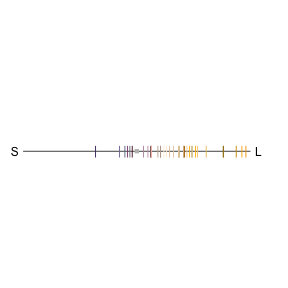For our trichromatic viewer we can use the honeybee Apis mellifera, one of the most significant and widespread pollinators. We'll also transform our quantum catches according to Fechner's law by specifying qcatch = 'fi', and will model photoreceptor stimulation under bright conditions by scaling our illuminant with the scale argument.

vis.flowers <- vismodel(flowers, visual = 'apis', qcatch = 'fi', scale = 10000)

tri.flowers <- colspace(vis.flowers, space = 'tri')


##                               s         m         l            x
## Goodenia_heterophylla 0.2882383 0.3587254 0.3530363 -0.004022828
## Goodenia_geniculata   0.2475326 0.3549832 0.3974842  0.030052720
## Goodenia_gracilis     0.1373117 0.3125304 0.5501579  0.168028001
## Xyris_operculata      0.2395035 0.3729820 0.3875145  0.010275990
## Eucalyptus_sp         0.2760091 0.3548096 0.3691813  0.010162377
## Faradaya_splendida    0.2654951 0.3431266 0.3913783  0.034119147
##                                 y    h.theta      r.vec
## Goodenia_heterophylla -0.05522995 -1.6435057 0.05537626
## Goodenia_geniculata   -0.10508396 -1.2922439 0.10929687
## Goodenia_gracilis     -0.24007647 -0.9601417 0.29303604
## Xyris_operculata      -0.11491764 -1.4816131 0.11537616
## Eucalyptus_sp         -0.07020755 -1.4270471 0.07093922


As in the case of our dichromat, the output contains relative photoreceptor stimulations, coordinates in the Maxwell triangle, as well as the 'hue angle' h.theta and distance from the origin (r.vec).

plot(tri.flowers, pch = 21, bg = spec2rgb(flowers))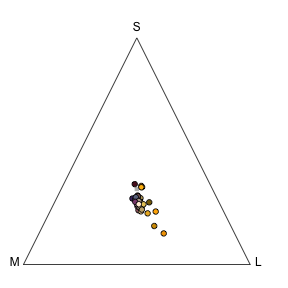Finally, we'll draw on the blue tit's visual system to model our floral reflectance spectra in a tetrahedral space, again using log-transformed quantum catches and assuming bright viewing conditions.

vis.flowers <- vismodel(flowers, visual = "bluetit", qcatch = "fi", scale = 10000)

tetra.flowers <- colspace(vis.flowers, space = "tcs")


##                               u         s         m         l         u.r
## Goodenia_heterophylla 0.2274310 0.2659669 0.2524170 0.2541851 -0.02256897
## Goodenia_geniculata   0.1625182 0.2721621 0.2828016 0.2825182 -0.08748184
## Goodenia_gracilis     0.0428552 0.2443091 0.3504414 0.3623943 -0.20714480
## Xyris_operculata      0.1766234 0.2709785 0.2642637 0.2881344 -0.07337659
## Eucalyptus_sp         0.2118481 0.2609468 0.2637023 0.2635028 -0.03815190
## Faradaya_splendida    0.1844183 0.2557660 0.2786393 0.2811764 -0.06558169
##                                s.r         m.r         l.r            x
## Goodenia_heterophylla  0.015966893 0.002417012 0.004185066 -0.007214866
## Goodenia_geniculata    0.022162064 0.032801599 0.032518179  0.006341799
## Goodenia_gracilis     -0.005690920 0.100441373 0.112394348  0.072312163
## Xyris_operculata       0.020978454 0.014263689 0.038134446  0.010505857
## Eucalyptus_sp          0.010946788 0.013702317 0.013502794  0.001565228
## Faradaya_splendida     0.005765955 0.028639328 0.031176408  0.015560661
##                                  y           z    h.theta     h.phi
## Goodenia_heterophylla -0.005415708 -0.02256897 -2.4976873 -1.190529
## Goodenia_geniculata    0.003861848 -0.08748184  0.5469755 -1.486123
## Goodenia_gracilis      0.033297418 -0.20714480  0.4315247 -1.203879
## Xyris_operculata      -0.010813615 -0.07337659 -0.7998327 -1.368146
## Eucalyptus_sp          0.001044768 -0.03815190  0.5885699 -1.521510
## Faradaya_splendida     0.007189965 -0.06558169  0.4328380 -1.315140
##                            r.vec     r.max r.achieved
## Goodenia_heterophylla 0.02430520 0.2692325 0.09027589
## Goodenia_geniculata   0.08779638 0.2508989 0.34992737
## Goodenia_gracilis     0.22191605 0.2678272 0.82857920
## Xyris_operculata      0.07490949 0.2552227 0.29350636
## Eucalyptus_sp         0.03819828 0.2503039 0.15260760


Tetrahedral data (via colspace(space = 'tcs')) may be visualised in an interactive plot by using tcsplot, along with the accessory functions tcspoints and tcsvol for adding points and convex hulls, respectively. As of version 1.0, pavo also includes a static tetrahedral plot. As with other colorspace plots there are a number of associated graphical options, though the theta and phi arguments are particularly useful in this case, as they control the orientation (in degrees) of the tetrahedron in the xy and yz planes, respectively.

When plotting the tetrahedral colorspace, one can also force perspective by changing the size of points relative to their distance from the plane of observation, using the arguments perspective = TRUE and controlling the size range with the range argument. Several other options control the appearance of this plot, you can check these using ?plot.colspace or ?tetraplot

plot(tetra.flowers, pch = 21, bg = spec2rgb(flowers), perspective = TRUE, range = c(1, 2), cex = 0.5)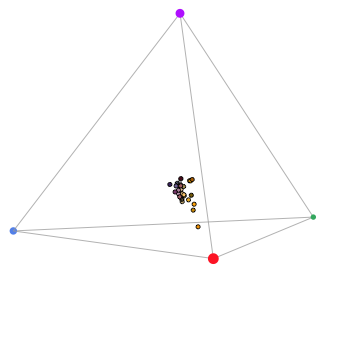Two additional functions may help with tetrahedral colorspace plotting:

• axistetra function can be used to draw arrows showing the direction and magnitude of distortion of x, y and z in the tetrahedral plot.
• legendtetra allows you to add a legend to a plot.
par(mfrow = c(1, 2), pty = "s")
plot(tetra.flowers, pch = 21, bg = spec2rgb(flowers))
axistetra(x = 0, y = 1.8)
plot(tetra.flowers, theta = 110, phi = 10, pch = 21, bg = spec2rgb(flowers))
axistetra(x = 0, y = 1.8)